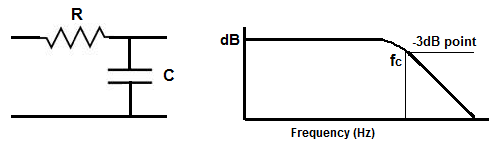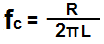﻿ -3dB Cutoff Frequency Calculator ﻿# -3dB Cutoff Frequency Calculator

RC -3dB Cutoff Frequency CalculatorThis -3dB cutoff frequency calculator calculates the -3dB cutoff point of the frequency response of a circuit, according to the formula,
fC=1/(2πRC). This is is the point in the response where the power reaches the halfway point; in other words, this is the point in a frequency response when the power gets cut in half, so there is half the power that there would be from the level that is present in the midband of the circuit. So, for example, if there is a sound of X volume present at full power. There will be X/2 power present at the 3db cutoff frequency.

This calculator is used for RC circuits, circuits consisting of a resistor and a capacitor.

A user enters the capacitance, C, and the resistance, R, and the result will automatically be calculated and shown. The frequency result which is displayed above is in unit hertz (Hz).

The cutoff frequency is a very valuable item to calculate because this value is important for all amplifier frequency responses. We must know the 3db cutoff frequency to see the range of frequencies where the amplifier doesn't amplify with full gain. Say if an amplifier has a cutoff frequency of 200Hz. At this point, we know that the gain that the amplifier produces is half of what it produces throughout the midband gain. At this point, the amplification drops significantly and doesn't amplify the signal well and with full gain, as it does with the midband gain. Thus, 3db cutoff frequency is used to find the cutoffs at both ends of an amplifier. This is why when you buy an amplifier at a store, it comes with a frequency response of 2 values, for example, 20Hz-18KHz. After these two ends, the amplifer cuts off and produces very low gain.

RL -3dB Cutoff Frequency CalculatorThis RL cutoff frequency calculator calculates the cutoff frequency point of the low pass filter, based on the values of the resistor, R, and inductor, L, of the circuit, according to the formula fc= R/(2πL).

To use this calculator, all a user must do is enter the inductance, L, of the inductor and the resistance, R, of the resistor. This calculator allows a user to select the magnitude of the henry of the capacitor, including picohenry (pH), nanohenry (nH), microhenry (µH), and henry (H). After the inductance and resistance values are entered, the user clicks the 'Calculate' button, and the result is automatically computed. The resultant value of the cutoff frequency calculated is in unit hertz (Hz).

Just as with the RC filter, the RL cutoff frequency calculator finds the cutoff frequency of the filter, which is the point in the frequency response of the circuit where the gain has been reduced by 3dB. This is crucial because it shows at which frequency the gain is cut in half (which is a 3dB drop). This point lets us know where in the filter the signals begin to attenuate greatly and no longer pass frequencies with very much gain. Thus, it shows the cutoff point of a filter.

As you can see in the above diagram, the low pass filter produces its full gain for low frequency signals and then begins producing lower gain signals. The cutoff frequency is the half power point, where the strength of the signal is now halved (half the strength).

Related Resources

﻿